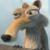## 用select查死数据库

yeisou 发布于 2011/09/27 10:33

0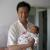0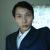```stmt = con.createStatement();
System.out.println(SQL);
rs = stmt.executeQuery(SQL);
int rowi = rowcol.getColumnCount();
int rownum = 0;
while (rs.next()) {
List<Object> lists = new ArrayList<Object>();
String Keyworkd = "";
String Values = "";
try{
for (int j = 1; j <= rowi; j++) {
try{
Keyworkd +=rowcol.getColumnName(j)+"";
Values += "?";
if(j!=rowi){
Keyworkd+=",";
Values+=",";
}
}catch(Exception e){System.out.println(e);}
}
if(rownum==1||rownum==100||rownum==200||rownum==300||rownum==400){
System.out.println("");
}
if(rownum==500){
rownum=0;
System.out.print(rowcount+",");
mssqlcon.commit();
}else{
System.out.print(rowcount+",");
}
rownum++;
INSERTSQL = "INSERT INTO  \"TestTable\" ("+Keyworkd+")VALUES("+Values+")";
INSERTSQL = StringUtils.replace(INSERTSQL, "'1000-", "'1900-");
//	System.out.println(INSERTSQL);
Object[] params = new Object[lists.size()];
int i=0;
for (Object obj : lists) {
params[i]=obj;
i++;
}
qr.update(mssqlcon, INSERTSQL, params);
rowcount++;
}catch (Exception e) {
System.out.println("\nErrMessage = "+e.getMessage()+"\n");
e.printStackTrace();
}
}```List转Object[] 早期都是自己写的方法，现在可以直接使用params.toArray()
0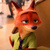0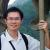1使用视图

2只允许查询部分视图

3屏蔽某些语句

0